# How To Create A Countdown Timer Using JavaScript

A countdown timer is an accurate timer that can be used for a website or blog to display the count down to any special event, such as a birthday or anniversary.
Basics of a countdown timer are :

1. Set a valid end date.
2. Calculate the time remaining.
3. Convert the time to a usable format.
4. Output the clock data as a reusable object.
5. Display the clock on the page, and stop the clock when it reaches zero.

Step 1 : Set a Valid End Date
The Valid end date and time should be a string in any of the formats understood by JavaScript’s Date.parse() method.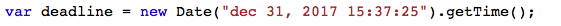Step 2 : Calculate Remaining Time
First we calculate the time remaining by subtracting the deadline by current date and time then we calculate the number of days,hours,minutes and seconds.The Math.floor() function is used to return the largest integer less than or equal to a given number.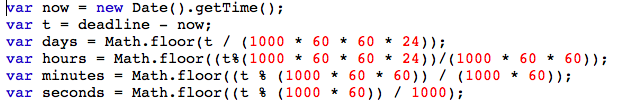Step 3 : Output the result
In the code below the result is given as output by id=”demo”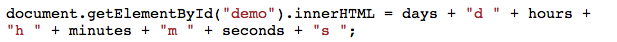Step 4 : Write some text if the countdown is over
If the countdown timer is over then “expired” will be displayed on the screen.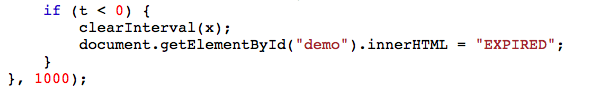INPUT :

 ` ` `<``html``> ` `<``head``> ` `<``style``> ` `p { ` `  ``text-align: center; ` `  ``font-size: 60px; ` `} ` ` ` ` ` `<``body``> ` `<``p` `id``=``"demo"``> ` `<``script``> ` `var deadline = new Date("Jan 5, 2018 15:37:25").getTime(); ` `var x = setInterval(function() { ` `var now = new Date().getTime(); ` `var t = deadline - now; ` `var days = Math.floor(t / (1000 * 60 * 60 * 24)); ` `var hours = Math.floor((t%(1000 * 60 * 60 * 24))/(1000 * 60 * 60)); ` `var minutes = Math.floor((t % (1000 * 60 * 60)) / (1000 * 60)); ` `var seconds = Math.floor((t % (1000 * 60)) / 1000); ` `document.getElementById("demo").innerHTML = days + "d "  ` `+ hours + "h " + minutes + "m " + seconds + "s "; ` `    ``if (t < ``0``) { ` `        ``clearInterval(x); ` `        ``document.getElementById("demo")``.innerHTML` `= ``"EXPIRED"``; ` `    ``} ` `}, 1000); ` ` ` ` `  ` ` ` `When the countdown is overCountdown Timer with CSS using JavaScript
INPUT:

 ` ` `<``html``> ` `<``head``> ` `<``style``> ` `body{ ` `    ``text-align: center; ` `    ``background: #00ECB9; ` `  ``font-family: sans-serif; ` `  ``font-weight: 100; ` `} ` `h1{ ` `  ``color: #396; ` `  ``font-weight: 100; ` `  ``font-size: 40px; ` `  ``margin: 40px 0px 20px; ` `} ` ` ``#clockdiv{ ` `    ``font-family: sans-serif; ` `    ``color: #fff; ` `    ``display: inline-block; ` `    ``font-weight: 100; ` `    ``text-align: center; ` `    ``font-size: 30px; ` `} ` `#clockdiv > div{ ` `    ``padding: 10px; ` `    ``border-radius: 3px; ` `    ``background: #00BF96; ` `    ``display: inline-block; ` `} ` `#clockdiv div > span{ ` `    ``padding: 15px; ` `    ``border-radius: 3px; ` `    ``background: #00816A; ` `    ``display: inline-block; ` `} ` `smalltext{ ` `    ``padding-top: 5px; ` `    ``font-size: 16px; ` `} ` ` ` ` ` `<``body``> ` `<``h1``>Countdown Clock ` `<``div` `id``=``"clockdiv"``> ` `  ``<``div``> ` `    ``<``span` `class``=``"days"` `id``=``"day"``> ` `    ``<``div` `class``=``"smalltext"``>Days ` `  `` ` `  ``<``div``> ` `    ``<``span` `class``=``"hours"` `id``=``"hour"``> ` `    ``<``div` `class``=``"smalltext"``>Hours ` `  `` ` `  ``<``div``> ` `    ``<``span` `class``=``"minutes"` `id``=``"minute"``> ` `    ``<``div` `class``=``"smalltext"``>Minutes ` `  `` ` `  ``<``div``> ` `    ``<``span` `class``=``"seconds"` `id``=``"second"``> ` `    ``<``div` `class``=``"smalltext"``>Seconds ` `  `` ` ` ` ` `  `<``p` `id``=``"demo"``> ` ` `  `<``script``> ` ` `  `var deadline = new Date("dec 31, 2017 15:37:25").getTime(); ` ` `  `var x = setInterval(function() { ` ` `  `var now = new Date().getTime(); ` `var t = deadline - now; ` `var days = Math.floor(t / (1000 * 60 * 60 * 24)); ` `var hours = Math.floor((t%(1000 * 60 * 60 * 24))/(1000 * 60 * 60)); ` `var minutes = Math.floor((t % (1000 * 60 * 60)) / (1000 * 60)); ` `var seconds = Math.floor((t % (1000 * 60)) / 1000); ` `document.getElementById("day").innerHTML =days ; ` `document.getElementById("hour").innerHTML =hours; ` `document.getElementById("minute").innerHTML = minutes;  ` `document.getElementById("second").innerHTML =seconds;  ` `if (t < ``0``) { ` `        ``clearInterval(x); ` `        ``document.getElementById("demo")``.innerHTML` `= ``"TIME UP"``; ` `        ``document.getElementById("day")``.innerHTML` `=``'0'``; ` `        ``document.getElementById("hour")``.innerHTML` `=``'0'``; ` `        ``document.getElementById("minute")``.innerHTML` `=``'0'` `;  ` `        ``document.getElementById("second")``.innerHTML` `= ``'0'``; } ` `}, 1000); ` ` ` ` ` ` `

OUTPUT :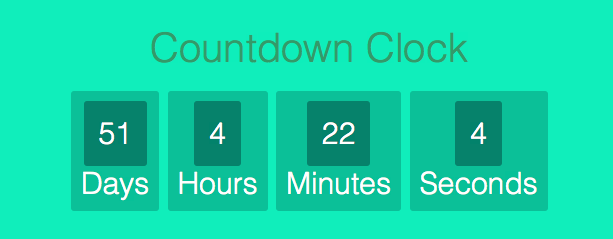When the Countdown Timer session is exceeded,the following output would be displayed: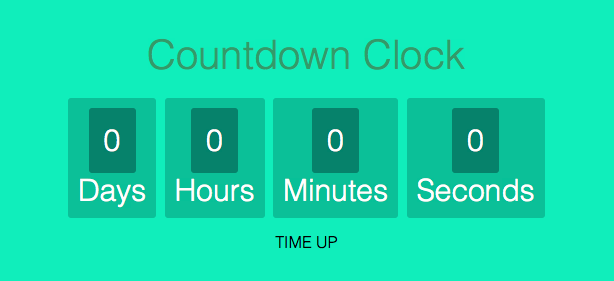Applications of Countdown Timer

• Used during Events to display the time left for its commencement.
• Used by online commerce websites to display time left for an ongoing sale.
• Used by websites during promotions
• Used in Car racing games,football games etc
• Used in Auction Websites to display the left for placing bids.

Benefits of making a countdown timer in JavaScript than using plugins

• The code will be lightweight because it will have zero dependencies.
• The website will perform better because there won’t be any need of loading external scripts and style sheets.
• The user gets more control because he has built the clock to behave exactly the way he wants it to rather than trying to bend a plugin according to his will.

This article is contributed by Shubrodeep Banerjee. If you like GeeksforGeeks and would like to contribute, you can also write an article using contribute.geeksforgeeks.org or mail your article to contribute@geeksforgeeks.org. See your article appearing on the GeeksforGeeks main page and help other Geeks.

My Personal Notes arrow_drop_upIf you like GeeksforGeeks and would like to contribute, you can also write an article using contribute.geeksforgeeks.org or mail your article to contribute@geeksforgeeks.org. See your article appearing on the GeeksforGeeks main page and help other Geeks.

Please Improve this article if you find anything incorrect by clicking on the "Improve Article" button below.

Article Tags :

2

Please write to us at contribute@geeksforgeeks.org to report any issue with the above content.